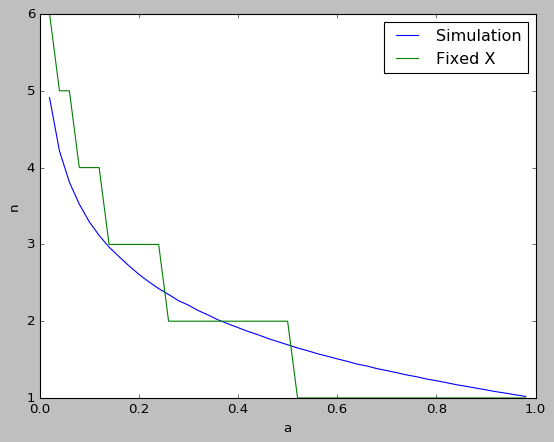#### 求期望

数学 概率论 随机过程    浏览次数：212        分享

3AlloAllo   2019-07-25 15:23

##### 1个回答
0

$$\lfloor 1-\log_2^a\rfloor$$

def GetN(a):
result = 0
for i in range(100000):
x = 
while a < x[-1]:
x.append(np.random.uniform(0, x[-1]))
result += ((len(x) - 1) / 100000)
return result
a = np.linspace(0.02, 0.98, 49)
expN = [GetN(i) for i in a]SofaSofa数据科学社区DS面试题库 DS面经我小宋   2019-09-10 09:55相关主题